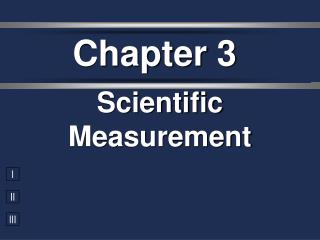DownloadDownload PresentationChapter 3

# Chapter 3

Download Presentation## Chapter 3

- - - - - - - - - - - - - - - - - - - - - - - - - - - E N D - - - - - - - - - - - - - - - - - - - - - - - - - - -
##### Presentation Transcript

1. Chapter 3 Scientific Measurement

2. Qualitative Gives results in a description, nonumerical form Quantitative Give results in a definite form, usually numbers and units Measurements

3. Accuracy vs. Precision • Accuracy - how close a measurement is to the accepted value • Precision - how close a series of measurements are to each other ACCURATE = CORRECT PRECISE = CONSISTENT

4. Accuracy vs. Precision

5. your value accepted value Percent Error • Indicates accuracy of a measurement

6. % error = 2.9 % Percent Error • A student determines the density of a substance to be 1.40 g/mL. Find the % error if the accepted value of the density is 1.36 g/mL.

7. Percent Error • A technician experimentally determined the boiling point of octane to be 124.1ºC. The actual boiling point of octane is 125.7ºC. Calculate the error and percent error. Error= 1.6ºC Percent error= 1.273 %

8. Significant Figures • Indicates precision of a measurement. • Recording Sig Figs • Sig figs in a measurement include the known digits plus a final estimated digit 2.31 cm

9. Significant Figures • Counting Sig Figs • All non-zero numbers are sig figs • Zeros depend on location in number: • LEADING zeros never count ---------0.0025 • EMBEDDED zeros always count---- 10,005 • TRAILING zeros only count if there is a point. --------------2,500 2,500. The underlined zeros do not count.

10. Significant Figures Counting Sig Fig Examples 1. 23.50 1. 23.50 4 sig figs 3 sig figs 2. 402 2. 402 3. 5,280 3. 5,280 3 sig figs 2 sig figs 4. 0.080 4. 0.080

11. Rounding • Need to use rounding to write a calculation correctly • Calculator gives you lots of insignificant figures and you must round to the right place • When rounding, look at the digit after the one you can keep • Greater than or equal to 5, round up • Less than 5, keep the same

12. Rounding • Round each measurement to 3 sig figs. • A. 87.073 meters • B. 4.3621 meters • C. 0.01552 meter • D. 9009 meters • E. 1.7777 meters • F. 629.55 meters 87.1meters 4.36 meters 0.0155 meter 9010 meters 1.78 meters 630 meters

13. Scientific Notation • Round each measurement to the number of significant figure shown in parenthesis. Write the answers in scientific notation. • A. 314.721 meters (4) • B. 0.001775 meter (2) • C. 64.32 X 10-1 meters (1) • D. 8792 meters (2) 3.147 X 102 1.8 108 60 X 10-1 8.8 X 103

14. Significant Figures • Calculating with Sig Figs (con’t) • Add/Subtract - The # with the lowest decimal value determines the place of the last sig fig in the answer. 224 g + 130 g 354 g 3.75 mL + 4.1 mL 7.85 mL 3.75 mL + 4.1 mL 7.85 mL  350g  7.9 mL

15. Significant Figures • C. 8.7 g + 15.43 g + 19 g = 43.13 g • D. 853.2 L – 627.443 L = 225.757 L • E. 5.47 m + 11m + 87.3000 m = 103.770m E. 5.47 11 + 87.3000 103.770 C. 8.7 15.43 + 19 43.13 D. 853.2 - 627.443 225.757 225.8 104 43

16. Significant Figures • Calculating with Sig Figs (con’t) • Exact Numbers do not limit the # of sig figs in the answer. UNLIMITED SIG FIGS • Counting numbers: 12 students • Exact conversions: 1 m = 100 cm • “1” in any conversion: 1 in = 2.54 cm

17. 3 SF Significant Figures • Calculating with Sig Figs • Multiply/Divide - The # with the fewest sig figs determines the # of sig figs in the answer. (13.91g/cm3)(23.3cm3) = 324.103g 4 SF 3 SF 324g

18. 1. (15.30 g) ÷ (6.4 mL)  2.4 g/mL 2 SF Significant Figures Practice Problems 4 SF 2 SF = 2.390625 g/mL  3700 a. 67 X 55 = 3685  0.30 d. 0.034 X 8.8 = 0.2992

19. Scientific Notation • Converting into Sci. Notation: • Move decimal until there’s 1 digit to its left. Places moved = exponent. • Large # (>1)  positive exponentSmall # (<1)  negative exponent • Only include sig figs. 65,000 kg  6.5 × 104 kg

20. 7. 2,400,000 g 8. 0.00256 kg 9. 7  10-5 km 10. 6.2  104 mm Scientific Notation Practice Problems 2.4  106 g 2.56  10-3 kg 0.00007 km 62,000 mm

21. Scientific Notation • Convert between scientific notation and regular notation *without changing the # of sig figs* • A. 4.61 X 10-4 • B. 3.69 X 106 • C. 0.000597 • D. 23,000,000 • E. 8.00 X 102

22. Scientific Notation • Calculating with Sci. Notation (5.44 × 107 g) ÷ (8.1 × 104 mol) = Type on your calculator: (5.44 X10 (8.1X10 7) 4) ÷ EE EE ENTER = 671.6049383 = 670 g/mol = 6.7 × 102 g/mol